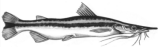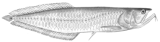# Abstracts of White PapersCategorical data tables can be modeled as finite, atomic, Boolean algebras. These models can be used to discover predictive rules in the data. The rules can be expressed by a Boolean algebraic formula in a normalized disjunctive form. The attributes and their values define a maximal algebra, and there exists a Boolean homomorphism from this to the algebra of actual data. The homomorphism kernel can be determined and transformed into a normalized disjunctive form. The predictive factors of all binary attributes can be derived immediately from this kernel. For attributes with more than two values a Boolean homomorphism can be constructed from the maximal algebra to the part of the table which contains the complement of the value to be determined. The predictive factors are in the subset, of the kernel, which matches the atoms which do contain the attribute-value. The Boolean meets which predict an attribute-value are partially or- dered, and this order can be determined by induction.

Keywords: Data Analytics, Categorical Data, Boolean Algebras, Induction

The white paper, 'Deriving Heuristic Rulse from Facts' (21 pages, 210K pdf, January 2007) is an informal treatment of the Emping 0.6 principles. The first section defines a fact model and a rule model in terms of partitions of a set. The second section treats the reduction algorithm and proves it finds all possible shortest rules. Therefore it produces a normal form representation of the rule model. The third section shows how to get the partial order, if there is one, of reduced antecedents through abduction. Entailment and overlap of underlying sets may allow further rule simplification. All examples use a data table from a classic paper by J.R. Quinlan, and the reductions were obtained with the Emping computer program.

The rest of content of the paper is, at this time (2012), judged to be incorrect and, at best, not very useful.Keywords: categorical data analysis, multivariate data analysis, data mining, rule based knowledge, machine learning